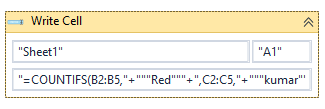# Trying to write a formula to excel cells

Hello All,
I am looking for some help writing a formula to a cell in an excel spreadsheet. I have been able to do this with simple formulas, so I know I am doing the procedure correct, and I know the formula works when I type it into an excel sheet.
However when I try to use the write cell function in uipath, I get a message saying the range doesn’t exist. If I delete this formula and type in a more simple one i.e. =J2+J3 it works fine. I think it may have something to do with the special characters in the formula. Any help would be appreciated. Here is the formula.

Extra points if you can figure out what the formula does lol

I cant recreate the error or try it out since the file isnt available. But have you tried using macros?

Would look something like this:
if its only for a single cell

``````Sub MacroForSingleCell()
End Sub
``````

For a range

``````Sub MacroForARange()
'Lastrow will have the range/row count from B column
Lastrow = Range("B" & Rows.Count).End(xlUp).Row
'We define a formula to be pasted from A2 to the last cell of range
End Sub
``````
1 Like

You can start trying out with a small formula. Hope below details help you.